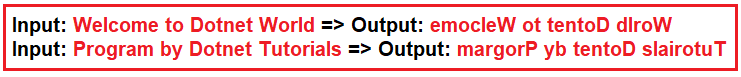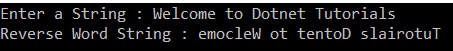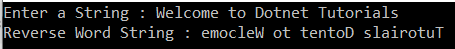# Reverse Each Word in a Given String in C#

## How to Reverse Each Word in a Given String in C#

In this article, I am going to discuss How to Reverse Each Word in a Given String in C# with some examples. Please read our previous article where we discussed How to Reverse a String in C# program with some examples. As part of this article, we are going to use the following three approaches to reverse each word in a given string C#.

1. Without using a built-in function
2. Using Stack
3. Using LINQ
##### Program Description:

The user will input a string and we need to reverse each word individually without changing its position in the string. Here, we will take the input as a string from the user and then we need to reverse each word individually without changing their position in the sentence as shown in the below image.##### Method1: Without using any built-in function:

In the following example, we generate all words separated by space. Then reverse the words one by one.

```using System;
using System.Collections.Generic;
using System.Text;

namespace LogicalPrograms
{
class Program
{
static void Main(string[] args)
{
Console.Write("Enter a String : ");

StringBuilder reverseWordString = new StringBuilder();
List<char> charlist = new List<char>();

for (int i = 0; i < originalString.Length; i++)
{
if (originalString[i] == ' ' || i == originalString.Length - 1)
{
if (i == originalString.Length - 1)
for (int j = charlist.Count - 1; j >= 0; j--)
reverseWordString.Append(charlist[j]);

reverseWordString.Append(' ');
charlist = new List<char>();
}
else
{
}
}
Console.WriteLine(\$"Reverse Word String : {reverseWordString.ToString()}");
}
}
}```

Output:##### Method2: Using Stack to Reverse Each Word in C#

Here, we are using a stack to push all words before space. Then as soon as we encounter a space, we empty the stack. The program is self-explained, so please go through the comments line.

```using System;
using System.Collections.Generic;

namespace LogicalPrograms
{
class Program
{
static void Main(string[] args)
{
Console.Write("Enter a String : ");

Stack<char> charStack = new Stack<char>();

// Traverse the given string and push all characters
// to stack until we see a space.
for (int i = 0; i < originalString.Length; ++i)
{
if (originalString[i] != ' ')
{
charStack.Push(originalString[i]);
}

// When seeing a space, then print contents of the stack.
else
{
while (charStack.Count > 0)
{
Console.Write(charStack.Pop());
}
Console.Write(" ");
}
}

// Since there may not be space after last word.
while (charStack.Count > 0)
{
Console.Write(charStack.Pop());
}

}
}
}
```
##### Method3: Using Linq to Reverse Each Word in C#
```using System;
using System.Linq;

namespace LogicalPrograms
{
class Program
{
static void Main(string[] args)
{
Console.Write("Enter a String : ");

string reverseWordString = string.Join(" ", originalString
.Split(' ')
.Select(x => new String(x.Reverse().ToArray())));

Console.WriteLine(\$"Reverse Word String : {reverseWordString}");
}
}
}
```

Output:##### Code Explanation:

Split the input string using a single space as the separator.

The Split method is used for returning a string array that contains each word of the input string. We use the Select method for constructing a new string array, by reversing each character in each word. Finally, we use the Join method for converting the string array into a string.

In the next article, I am going to discuss How to Remove Duplicate Characters from a given string in C# using different mechanisms. I hope now you understood How to Reverse Each Word in a Given String in C# with different mechanisms.

### 3 thoughts on “Reverse Each Word in a Given String in C#”

1.public class ReverseEachWord
{
public static void Main(String[] args)
{
ReverseWord(a);
}

public static void ReverseWord(string a)
{
string newString = string.Empty;

for (int i = a.Length-1; i >=0; i–)
{
newString = newString + a[i];

}
Console.WriteLine(newString);
}
}

2.// A simple attempt from my side 🙂 //

public class Program
{
public static void Main()
{
string str = “dotnettutorials rocks”;
var spcArr = str.Split(‘ ‘);
str = “”;
foreach (var w in spcArr)
{
char[] arr = w.ToCharArray();
for (int i = arr.Length – 1; i >= 0; i–)
{
str += arr[i];
}

str += ” “;
}

Console.WriteLine(str.Trim());
}
}

3.static void Main()
{
string[] g = a.Split(‘ ‘);
string reverse = “”;
string[] q = new string;
foreach (var h in g)
{
int i = 0;
q[i] = h;
foreach (char ch in q[i])
reverse = ch + reverse;
Console.Write(reverse+” “);
reverse = “”;
i++;
}
}